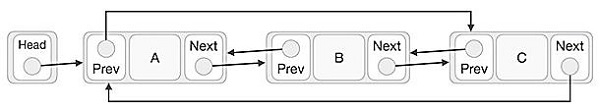# Doubly Linked Circular Lists in C++

Circular Linked List is a variation of Linked list in which the first element points to the last element and the last element points to the first element. Both Singly Linked List and Doubly Linked List can be made into a circular linked list.

In doubly linked list, the next pointer of the last node points to the first node and the previous pointer of the first node points to the last node making the circular in both directions.As per the above illustration, following are the important points to be considered.

• The last link's next points to the first link of the list in doubly linked list.

• The first link's previous points to the last of doubly linked list.

## Algorithm

displayForward():
Begin
while ptr := null, do
print ptr ->key, ptr ->data
ptr := ptr -> next
done
End
displayBackward():
Begin
ptr := last
while ptr := null, do
print ptr ->key, ptr ->data
ptr := ptr -> prev
done
End
insertFirst(key, data):
Begin
create new node with key and data
if list is empty, then
last := node
else
end if
End
insertLast (key, data):
Begin
create new node with key and data
if list is empty, then
last := node
else
last -> next := node
node -> prev:= last
end if
last := node
End
insertAfter(key, newKey, data):
Begin
if head is null, then return false
while key of current is not key, do
if current -> next is not null, then
return false
else
current := next of current
end if
done
create new node with newKey and data
if current = last, then
next of node := null
last := node
else
next of node := next of current
prev of next of current := node
end if
prev of node := current
next of current := node
return true;
End
deleteFirst():
Begin
if next of head is null, then
last := null
else
prev of next of head := null
end if
return tempNode
End
deleteLast():
Begin
tempNode := last
if next of head is null, then
else
next of prev of last := null
end if
last := prev of last
return tempNode
End
deleteNode(key):
Begin
curr := head and prev := null
return null
end if
while key of currr is not same as key, do
if next of curr is null, then
return null
else
prev := curr
curr := next of curr
end if
done
if curr is head, then head := next of head, otherwise next of prev of curr = next of curr
if curr is last, then last := prev of curr, otherwise prev of next of curr = prev of curr
return curr
End

## Example

Live Demo

#include <iostream<
using namespace std;
class node {
public:
int data;
int key;
node *next;
node *prev;
};
node *last = NULL;
node *current = NULL;
//is list empty
bool isEmpty() {
}
int length() {
int length = 0;
node *current;
for(current = head; current != NULL; current = current->next){
length++;
}
return length;
}
//display the list in from first to last
void displayForward() {
//start from the beginning
//navigate till the end of the list
printf("\n[ ");
while(ptr != NULL) {
printf("(%d,%d) ",ptr->key,ptr->data);
ptr = ptr->next;
}
printf(" ]");
}
//display the list from last to first
void displayBackward() {
//start from the last
node *ptr = last;
//navigate till the start of the list
printf("\n[ ");
while(ptr != NULL) {
//print data
printf("(%d,%d) ",ptr->key,ptr->data);
//move to next item
ptr = ptr ->prev;
}
}
//insert link at the first location
void insertFirst(int key, int data) {
if(isEmpty()) {
}
else {
}
//point it to old first link
//point first to new first link
}
//insert link at the last location
void insertLast(int key, int data) {
if(isEmpty()) {
}
else {
//mark old last node as prev of new link
}
//point last to new last node
}
//delete first item
node* deleteFirst() {
last = NULL;
}
else {
}
}
//delete link at the last location
node* deleteLast() {
}
else {
last->prev->next = NULL;
}
last = last->prev;
}
//delete a link with given key
node* del(int key) {
node* previous = NULL;
//if list is empty
return NULL;
}
//navigate through list
while(current->key != key) {
//if it is last node
if(current->next == NULL) {
return NULL;
}
else {
previous = current;
current = current->next;
}
}
//found a match, update the link
//change first to point to next link
}
else {
current->prev->next = current->next;
}
if(current == last) {
//change last to point to prev link
last = current->prev;
}
else {
current->next->prev = current->prev;
}
return current;
}
bool insertAfter(int key, int newKey, int data) {
//if list is empty
return false;
}
//navigate through list
while(current->key != key) {
//if it is last node
if(current->next == NULL) {
return false;
}
else {
current = current->next;
}
}
if(current == last) {
}
else {
}
return true;
}
main() {
insertFirst(1,10);
insertFirst(2,20);
insertFirst(3,30);
insertFirst(4,1);
insertFirst(5,40);
insertFirst(6,56);
printf("\nList (First to Last): ");
displayForward();
printf("\n");
printf("\nList (Last to first): ");
displayBackward();
printf("\nList , after deleting first record: ");
deleteFirst();
displayForward();
printf("\nList , after deleting last record: ");
deleteLast();
displayForward();
printf("\nList , insert after key(4) : ");
insertAfter(4,7, 13);
displayForward();
printf("\nList , after delete key(4) : ");
del(4);
displayForward();
}

## Output

List (First to Last):
[ (6,56) (5,40) (4,1) (3,30) (2,20) (1,10) ]
List (Last to first):
[ (1,10) (2,20) (3,30) (4,1) (5,40) (6,56)
List , after deleting first record:
[ (5,40) (4,1) (3,30) (2,20) (1,10) ]
List , after deleting last record:
[ (5,40) (4,1) (3,30) (2,20) ]
List , insert after key(4) :
[ (5,40) (4,1) (7,13) (3,30) (2,20) ]
List , after delete key(4) :
[ (5,40) (7,13) (3,30) (2,20) ]

Updated on: 10-Aug-2020

1K+ Views